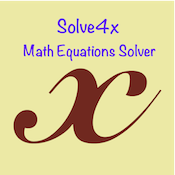## Solve4xSolve4x – The math equation Solver,our new educational utility app for iPhone. Solve4x is designed for middle and high school students and can solve basic Linear equations (Up to 3 variables), Quadratic and Cubic equations. The students can type in the equations using the keyboard or they can even take a picture of the equation using the iPhone inbuilt camera. The app also solves 2×2 and 3×3 matrices (such as finding determinant or matrix inverse). Solve4x is free to download from Apple App store.

Some of the types of equations that can be solved are:-
a) Linear Equation in one variable such as
X – 5X – 3x + 15X = -16

b) Linear Equation in two variables such as
M + N = 10
2M – 3N = 0

c) Linear Equation in Three variables such as
A + B + C = 3
3A – B – C = 1
-A + B + 5C = 1

d) Quadratic equation in one variable such as
k^2 + 16k + 64 = 0

e) Cubic equation in one variable such as
z^3 + 2z^2 + 3z + 1 = 7

Salient features of the app:-
– Equations can be entered using the keyboard or you can use the Snap feature. With the snap feature the equations can be captured from say a text book using the inbuilt iPhone Camera.
– Fast and Offline solution of the equation. The equation solution engine uses the native iOS capabilities and hence it is real fast. No internet needed to solve the equations. Most of the equations are solved within seconds.
– An option to email the solution of the equations from within the app itself.
– Silent app, no noise, no cartoons
– No social media interaction